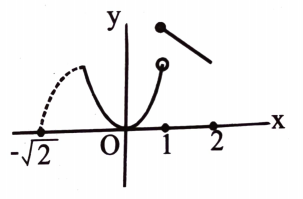Maxima and minima
Question

# If now match the following Column IColumn IIA. The number of critical points of f(x) in [-2, 2] isP. 1B. The length of the longest interval in which f(x) satisfies Rolle's theorem isQ. 2C.The number of values of ‘c’ of Rolle's Mean value theoremR. 3D. The maximum value of f(x) in [-2, 2] is S. 0

Difficult
Solution

##Get Instant Solutions